# Water mathematics

You have seen many different kinds of water in your life already, but not everyone knows what makes natural water look realistic. Different natural water types have several properties in common but they are absolutely different in others. The key ingredients of natural waters are:

• Reflecting the objects visible above the surface
• Refracting the objects under the surface
• Multiple reflections and refraction
• Appropriate ratio between reflection and refraction: the Fresnel term
• Some small modification in the colors (dirtier water), water-fog
• Moving surface
• Specular reflection
• Deep water phenomena including caustics, spray, Godrays, foam and the Kelvin wedge

## Reflection

Reflection is the phenomena when the wave front changes direction at an interface between two different media, in the way that the wave front returns into the medium from which it originated. In some aspects the surface of the water acts like a perfect mirror. Electromagnetic waves of light are reflected on the surface. The physical law for this: the angle of reflection equals the angle of incidence, and we measure these angles to the normal-vector of the surface, namely the two angles α and β are equal:Taking into consideration only the reflection behavior of the water it is easy to calculate the color of the pixels on the water surface. Mirroring the position of the camera to the plane of the surface gives the exact location of the virtual view: just determine which color has the object which is visible through every pixel of the water from the virtual view. This idea is shown on the next figure:

If the camera is in point A, the perceived color on the water surface will be the color of the object visible from point B through the same intersection point. Point B is exactly the same far from the plane of the water as point A – the two distances are marked with letter “k” on the figure.

## Refraction

The speed of electromagnetic waves is different in different media. The change happening when it passes from one medium to another causes the phenomenon of refraction. The Snell’s law (named after the Dutch mathematician Willebrord Snellius) describes the relationship between the angle of incidence and refraction: the ratio between them is a constant depending on the media or more exactly the ratio of the sines of the angles equals the ratio of velocities in the two media: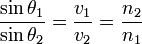or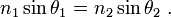These angles are measured in respect to the normal-vector of the boundary between the two media.

According to this law the direction of the wave can be refracted towards or from the normal line, depending on the relative refraction-indices of the media. The next figure shows an example:In this case the velocity is lower in the second medium (V2<V1), the ray in the second medium is closer to the normal-line.
The index of refraction for air is 1, for water is 4/3.

## The third dimension

The previously discussed examples and definitions were only two-dimensional, but for the real world, everything needs to be formed into 3D. The following equations describe the direction after refraction in 3D. For explanation, see [MFGD]. Let s be the incoming ray of light (vector), t the transformed ray of light, n the normal-vector of the surface, η1 and η2 are the velocity indices. The transformed vector has two components: one parallel and one perpendicular to n. This can be written in the following form:

t = –n cos(θ2) + m sin(θ2)

To calculate the two coefficients, we need to use the fact that only the angle along the surface changes, and not the entire direction. m can be defined as follows:

m = perpns / sin(θ1) = s – (ns) n / sin(θ1)
Using the previous equations [MFGD] described the following result:This equation contains the possibility to have negative square root, which means that the equation is not defined for every angles and coefficients. The physical reason for this is described in the following paragraph.

## Critical angle

There is one more important phenomenon that I need to mention in connection with refraction. If the light is coming from a media with lower velocity, the angle will change from the normal, so the angle between the normal and the beam will be bigger in the target media. This means that to get 90 degree in the target medium a smaller angle is enough in the source medium. This specific angle is called critical angle. The critical and any higher angle results a refraction vector which is parallel to the surface of the media. If this happens, the wave will be refracted along the border of the media, it will not intrude the target medium, the so-called total internal reflection will occur. This critical angle is about 50 degree at a water-air boundary.The sources of these illustrative images are Wikipedia and http://www.glenbrook.k12.il.us.
There is a funny flash application to play with the reflective-refractive angles and media on this site: http://www.ps.missouri.edu/rickspage/refract/refraction.html

## Multiple reflection and refraction

The light beams are reflected and refracted on the surface of the water, but to a certain amount the transformed light beams meat the air-water border again and reflection and refraction happens newly. This is illustrated on the next figure:The source of the image is [TEoNIaRT].

## The reflection-refraction ratio: the Fresnel term

The first two sections described reflection and refraction. They both happen to electromagnetic waves on the border of different media like on the next figures: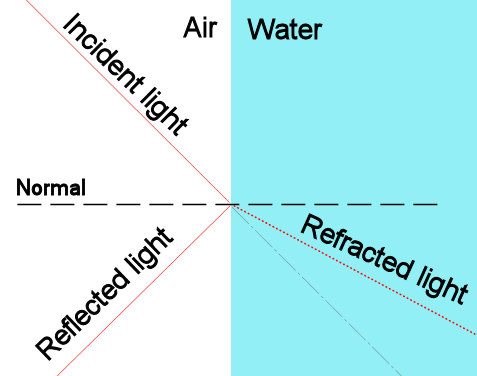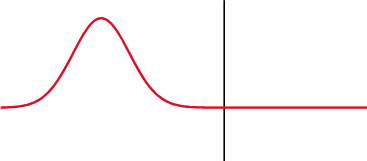But how to get the accurate ratio between reflection and refraction? Augustin-Jean Fresnel (/freɪˈnɛl/) worked out the laws of optics in the early 19th century. His equations give the degree of reflectance and transmittance at the border of two media with different density. Derivation of them is outside the scope of this paper.

The wave has two components: a parallel and a perpendicular. Ei is the amplitude of the incident wave,Er and Et are the amplitudes of the reflected and the transmitted wave. θi, θr and θt are the angles between the surface normal and the beam of incidence, refraction and transmittance.

#### r = Er/Ei t = Et/Ei

For the perpendicular component the equations are the following:

#### r = [nicos(θi)− ntcos(θt)]/[nicos(θi) + ntcos(θt)] t = [2nicos(θi)]/[nicos(θi) + ntcos(θt)]

The next equations are showing the properties of the parallel components:

#### r = [nicos(θi)− ntcos(θi)]/[nicos(θt) + ntcos(θi)] t = [2nicos(θi)]/[nicos(θt) + ntcos(θi)]

The more elegant version of them are:

#### rperpendicular = −[sin(θi)−sin(θt)]/[sin(θi)+sin(θt)]

and

rparallel =[tan(θi)−tan(θt)]/[tan(θi)+tan(θt)]

If the light is polarized to have only perpendicular component, we call it S-polarized. Similarly, if it has only parallel components, it is called P-polarized. The next figure shows example coefficients depending on the angles for both S and P polarized cases: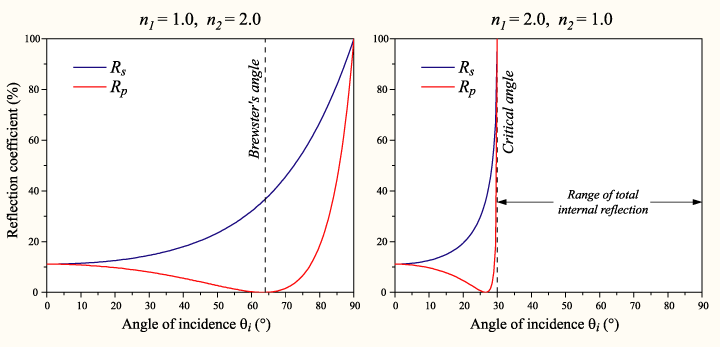As visible on the figure, in case of moving from denser medium to a less dense one (on the right), the reflection coefficient is 1 above the critical angle. This phenomenon is known as total internal reflection, as metioned earlier.

http://en.wikipedia.org/wiki/Fresnel_term

http://hyperphysics.phy-astr.gsu.edu/Hbase/phyopt/freseq.html

http://www.inyourfacefotos.com/fresnel.htm

## Moving water

Describing ocean waves is a huge challenge. It has several different components and their cooperation results in a very complex system. Basically there are two different kinds of mechanical wave motion: longitudinal and transverse. The direction of oscillation relative to the wave motion distinguishes them. If the oscillation is parallel to the wave motion it is called longitudinal wave: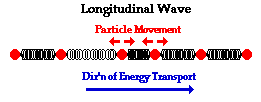If the oscillation is perpendicular to the wave motion it is called transversal wave: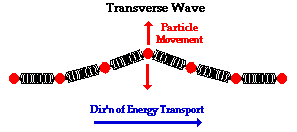Examples are, for example, sound wave in air for longitudinal waves and the motion of a guitar-string if you play the guitar for transverse waves. For both kinds of waves amplitude is the maximum displacement of a wave from the equilibrium and wavelength is the shortest length between two points of the wave which are in the same wave-phase. The frequency shows the number of wave cycles in a second. It is easy to calculate the speed of a wave from these data: wave speed equals the product of frequency and wavelength.

The blowing wind and gravity together forms the ocean waves which propagate along the surface of the water and air. This compound system has both longitudinal and transverse components and makes water particles move in a circular path. The closer to the surface a particle is, the bigger the radius of the motions becomes. This kind of wave is called surface wave and is illustrated on the next figure:At point A, where the water is deeper, the path is circle, at point B, where the water is shallow, the path of the motion becomes elliptic with decreasing water depth. The arrow #1 shows the direction of propagation. #2 shows the crest of the wave, #3 shows the wave trough.

The next animation tries to visualize the process:The source of the image is the homepage of Kettering University.

The following equation describes the dispersion relationship of the surface waves (the source is the scienceworld homepage):where ω is the angular frequency, g is the gravitational acceleration, k is the wavenumber, γ is the surface tension, and ρ is the density. Solving for ω gives## Compound systems – summation of different waves

The previously discussed theoretical background is enough to describe the motion of ocean waves, but we need to use more components with different amplitudes and wavelengths to get a more realistic result: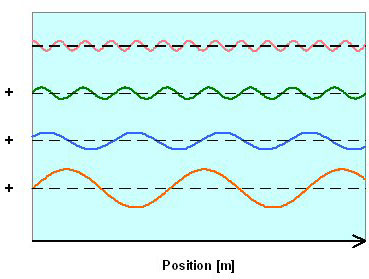The sum of the components results the next wave: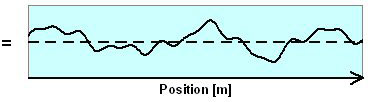In the tree-dimensional world different components have not only different amplitudes and wavelength, but different directions as well. The dominant direction will be the one with bigger amplitude and longer period. The next figure shows three components:And the sum of the components can approximate the real ocean surface realistic:http://oceanworld.tamu.edu/resources/ocng_textbook/chapter16/chapter16_01.htm

## Gerstner Waves

A Czech scientist, Jozef Gerstner obtained the first exact solution describing water waves of arbitrary amplitudes in 1802. His model also describes the cycloidal movement of the surface waves. In this model the water depth is large compared to the wave length. The resulted curve is also called trachoid.

Displacements are defined with the following equations:

x = X0-(k/k0) * A sin( k * X0 – ωt)
y = A cos( k * X0 – ωt)

where X0 is the undisturbed surface point, A is the wave amplitude, k is the wave vector and k0 is the magnitude.

Gerstner waves become close to sinusoidal if the amplitudes are very small, but they break if the amplitudes are bigger: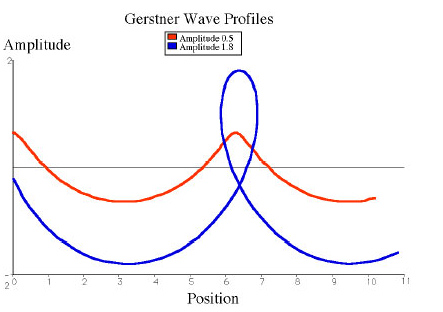These qualities allow Gerstner waves to describe various surface waves under different conditions.

For more details, see [IAoOW] or [GW].

## The Navier-Stokes Equations

Navier-Stokes Equations (NSE) are nonlinear partial differential equations and describe the motion of incompressible viscose fluids. In NSE there are three types of forces acting:

• Gravity: Fg = ρG, where ρ is the density and G is the gravitational force (9.81 m/s2).
• Pressure forces: These forces act inwards and normal to the water surface.
• Viscose forces: These are forces due to friction in the water and acts in all directions on all elements of the water.

The time dependant chaotic, stochastic behavior of fluids is called turbulence. Navier-Stokes equations are thought to describe the phenomena, but it is not answered yet, how to decide whether smooth, physically reasonable solutions exist for the equations. Actually a 1,000,000 dollar prize is offered to whoever makes preliminary progress toward a mathematical theory which will help in the understanding of this phenomenon. Further discussion is outside the scope of this paper. The Navier-Stokes equations are given by: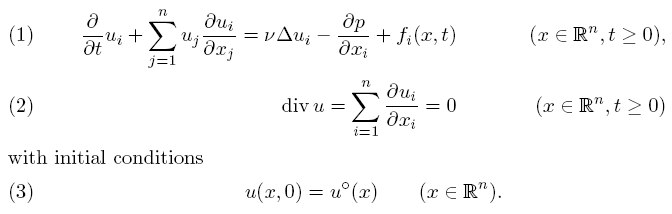Where, uº(x) is a given, C divergence-free vector field on Rn, fi(x, t) are the components of a given, externally applied force (e.g. gravity), ν is a positive coefficient (the viscosity), and is the Laplacian in the space variables. For more details, see [NSEP], [FDfP] or read about an implemented version: [GPUGEMS].

## Specular lights

Materials having a flat surface (e.g.: leather, glass and water also) present an interesting phenomenon which I have not mentioned yet. There are several different reflection models, some of them make the created image much more realistic while others help to improve the smaller details. One of these in the second group is specular reflection.

Materials like sand have irregular, bumpy surface and this makes incoming light to be reflected in every directions. This is shown on the next figure: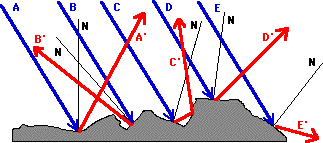But if the material has flat surface, the light waves will be parallel after reflection as well. Because of this property shiny, bright spots will be formed for example on the leather, on different metals or on the water if it is illuminated from a certain angle. The Phong illumination model describes this in the way which is most commonly used in 3D computer graphics. Phong Bui Tong developed his model in 1975 and it is still very popular. According to this model the intensity of point has three components: an ambient, a diffuse and a specular component and the specular highlight is seen when the viewer is close to the direction of reflection. The intensity of this kind of light falls off sharply when the viewer moves away from the direction of specular reflection.Vector L points towards the light-source, V towards the viewer, N is the normal-vector of the surface, R is the direction of reflection while H halves the angle between L and V.

The approximation of the falloff of the intensity in the Phong model uses the power of the cosine of the angle. The specular part of the original formula looks like this:
kspec cosn(β)

where β is the angle between R and V; kspec is the specular coefficient. The exponent n can influence the sharpness of the falloff. A bigger exponent can describe a shinier surface with less gentle falloff. The dot product of two vectors equals the cosine of the angle between them, so the formula can be written in the following form:

kspec (V·N)n

Where “·” is the dot product.

With the diffuse reflection model the Phong illumination model is the following (I means intensity):

I = kambient * Iambient + (Ip /(d)) [kdiffuse * (N·L) + kspecular * (V·R)n]

Where Is are the different intensities, ks are the ambient, diffuse and specular coefficients, and · is the dot product. Namely, the intensity of a point is equal to the sum of the ambient light-intensity and the sum of the diffuse and specular intensity scaled to the distance of the light-source.

http://www.dgp.toronto.edu/~karan/courses/csc418/fall_2002/notes/illum_local.html

http://www.mini.pw.edu.pl/~kotowski/Grafika/IlluminationModel/Index.html

## Deep water phenomena

#### Caustics

A wavy water surface presents caustics: the light beams are refracted in very choppy directions, the coincidence of rays intensifies, leading to very bright regions on every surface under the water: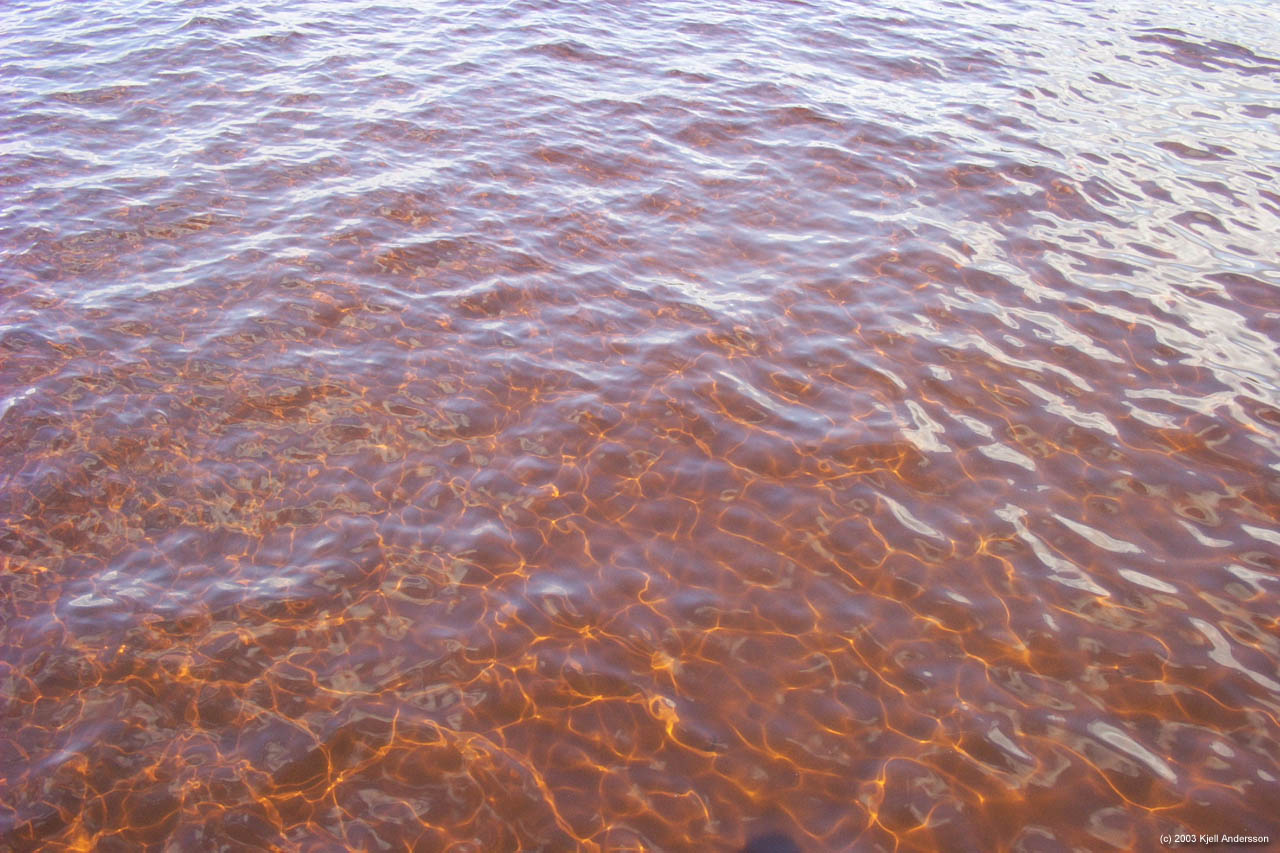Formation of caustics is illustrated on the next figure: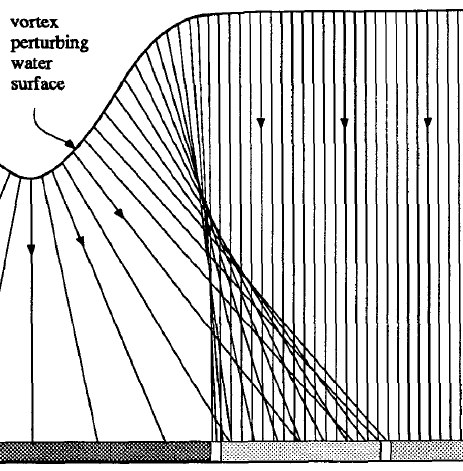Caustics are bright regions where the light beams coincident

#### Godrays

The same physical effects which cause caustics can create Godrays (described in [DWAaR]). The changing water surface focuses and defocuses light rays. The small particles floating in the water can get into these focus points and become visible for a short period. The continuously changing patterns created by these effects are called Godrays which are visible if you are looking from underwater towards the light source. A rendered example is shown on the next figure: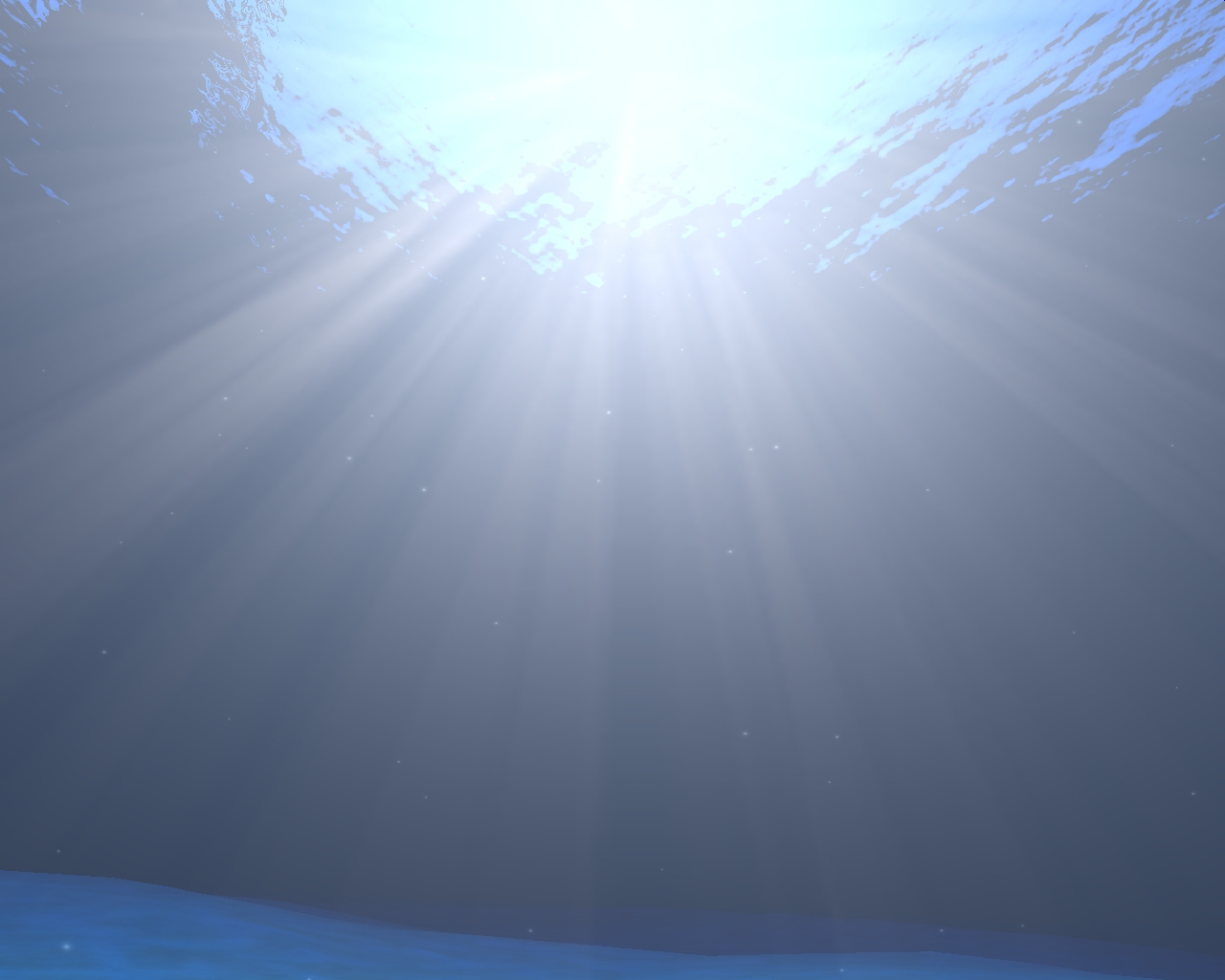Godrays – The image was created by the Typhoon engine. [TYPHOON]

#### Whitecaps and foam

Breaking waves produce foam, and the scummy parts of the breaking waves are called whitecaps. According to [RNW], the area of whitecaps depends on the temperature of the water and the air and on water chemistry. They used an empirical formula to approximate the fraction of the water covered by foam that modifies optical properties on the water’s surface:

f = 1.59 * 10-5 U2.55 exp[0.086 * (Tw – Ta )]

Where f is the fractional area, U is the wind speed, Tw and Ta are the water and air temperature in degrees Celsius.

#### The Kelvin wedge

On open water, moving ships generate waves. These waves cannot be in any reasonable approximation treated as exclusively longitudinal. The phenomenon, the so-called Kelvin wedge was first analyzed by Lord Kelvin. An ideal example is visualized on the next figure: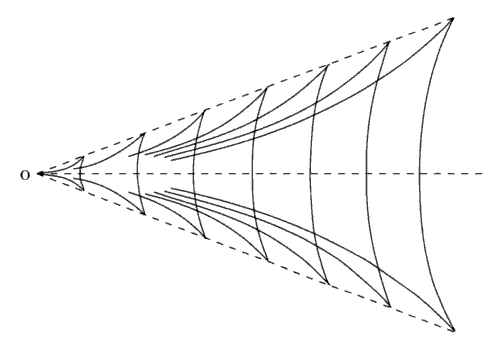The complicated wave pattern behind a ship is influenced by the viscosity of the water, by the moving directions, gravity, not to mention non-linear effects which become significant when the amplitude is large. Stern waves and bow waves are superposed on one another, and not infrequently, other wave systems may be discerned originating from somewhere between the bow and stern. The fact, that the angle enclosed by the Kelvin wedge is independent of the speed of the boot can be surprising, but the explanation is out of the scope of this paper. For more details, see [FDfP].

## References

[MFGD] – Mathematics for Game Developers – Christopher Tremblay – Thomson course technology.

[TYPHOON] – Typhoon 3D engine

[GW] – Jefrey Alcantara: Gerstner waves

## Microsoft Word doc version